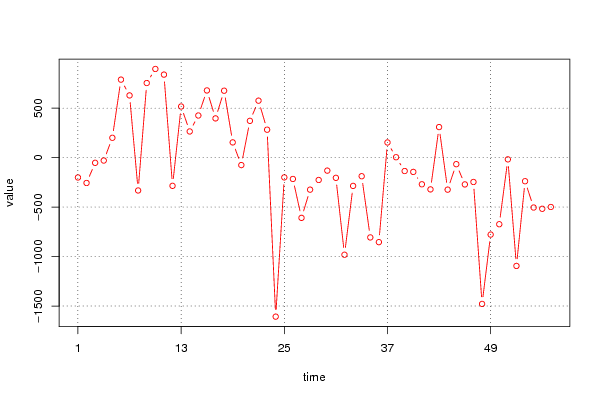## Free Statistics

of Irreproducible Research!

Author's title
Author*The author of this computation has been verified*
R Software Modulerwasp_univariatedataseries.wasp
Title produced by softwareUnivariate Data Series
Date of computationThu, 25 Nov 2010 20:21:08 +0000
Cite this page as followsStatistical Computations at FreeStatistics.org, Office for Research Development and Education, URL https://freestatistics.org/blog/index.php?v=date/2010/Nov/25/t1290716431mbbm7hcvvhxpbhb.htm/, Retrieved Sat, 23 Sep 2023 16:58:51 +0000
Statistical Computations at FreeStatistics.org, Office for Research Development and Education, URL https://freestatistics.org/blog/index.php?pk=101501, Retrieved Sat, 23 Sep 2023 16:58:51 +0000
QR Codes:Original text written by user:
IsPrivate?No (this computation is public)
User-defined keywords
Estimated Impact162
Family? (F = Feedback message, R = changed R code, M = changed R Module, P = changed Parameters, D = changed Data)
-     [Univariate Data Series] [Arabica Price in ...] [2008-01-05 23:43:18] [74be16979710d4c4e7c6647856088456]
- RMPD    [Univariate Data Series] [Mini-Tutorial] [2010-11-25 20:21:08] [6427096bd21c899f2c90594929aeeec2] [Current]
-   PD      [Univariate Data Series] [Mini-Tutorial (Un...] [2010-11-25 20:59:07] [845827b7f02503df17c96f445745fee7]
Feedback Forum

Post a new message
Dataseries X:
-200.5
-256
-52.3
-29.1
200.6
788.5
629.1
-331.8
754.1
896.5
839.3
-284.9
516.8
264.8
426
679.4
396.9
676.4
154.2
-75.3
371.8
575.9
282.4
-1607.2
-199.4
-215.5
-608.5
-323.5
-225.9
-131.2
-204
-981.4
-285.7
-187.8
-806.4
-854.1
153.5
4.3
-135.5
-144.2
-269.1
-320.8
309.1
-323.4
-65.9
-270.6
-246
-1478.7
-777.6
-673
-17.4
-1094.6
-238.1
-504.2
-517.1
-498.8


 Summary of computational transaction Raw Input view raw input (R code) Raw Output view raw output of R engine Computing time 2 seconds R Server 'Gwilym Jenkins' @ 72.249.127.135

\begin{tabular}{lllllllll}
\hline
Summary of computational transaction \tabularnewline
Raw Input & view raw input (R code)  \tabularnewline
Raw Output & view raw output of R engine  \tabularnewline
Computing time & 2 seconds \tabularnewline
R Server & 'Gwilym Jenkins' @ 72.249.127.135 \tabularnewline
\hline
\end{tabular}
%Source: https://freestatistics.org/blog/index.php?pk=101501&T=0

[TABLE]
[ROW][C]Summary of computational transaction[/C][/ROW]
[ROW][C]Raw Input[/C][C]view raw input (R code) [/C][/ROW]
[ROW][C]Raw Output[/C][C]view raw output of R engine [/C][/ROW]
[ROW][C]Computing time[/C][C]2 seconds[/C][/ROW]
[ROW][C]R Server[/C][C]'Gwilym Jenkins' @ 72.249.127.135[/C][/ROW]
[/TABLE]
Source: https://freestatistics.org/blog/index.php?pk=101501&T=0

Globally Unique Identifier (entire table): ba.freestatistics.org/blog/index.php?pk=101501&T=0

As an alternative you can also use a QR Code:

The GUIDs for individual cells are displayed in the table below:

 Summary of computational transaction Raw Input view raw input (R code) Raw Output view raw output of R engine Computing time 2 seconds R Server 'Gwilym Jenkins' @ 72.249.127.135

 Univariate Dataseries Name of dataseries Waarde handelsbalans Intra-EU Source Description Number of observations 56

\begin{tabular}{lllllllll}
\hline
Univariate Dataseries \tabularnewline
Name of dataseries & Waarde handelsbalans Intra-EU \tabularnewline
Source &  \tabularnewline
Description &  \tabularnewline
Number of observations & 56 \tabularnewline
\hline
\end{tabular}
%Source: https://freestatistics.org/blog/index.php?pk=101501&T=1

[TABLE]
[ROW][C]Univariate Dataseries[/C][/ROW]
[ROW][C]Name of dataseries[/C][C]Waarde handelsbalans Intra-EU[/C][/ROW]
[ROW][C]Source[/C][C][/C][/ROW]
[ROW][C]Description[/C][C][/C][/ROW]
[ROW][C]Number of observations[/C][C]56[/C][/ROW]
[/TABLE]
Source: https://freestatistics.org/blog/index.php?pk=101501&T=1

Globally Unique Identifier (entire table): ba.freestatistics.org/blog/index.php?pk=101501&T=1

As an alternative you can also use a QR Code:

The GUIDs for individual cells are displayed in the table below:

 Univariate Dataseries Name of dataseries Waarde handelsbalans Intra-EU Source Description Number of observations 56PNG link Postscript link PDF link

Parameters (Session):
par1 = Waarde handelsbalans Intra-EU ; par4 = 12 ;
Parameters (R input):
par1 = Waarde handelsbalans Intra-EU ; par2 = ; par3 = ; par4 = 12 ;
R code (references can be found in the software module):
if (par4 != 'No season') {par4 <- as.numeric(par4)if (par4 < 4) par4 <- 12}summary(x)n <- length(x)bitmap(file='test1.png')if (par4=='No season') {plot(x,col=2,type='b',main=main,xlab=xlab,ylab=ylab,xaxt='n')axis(1,at=seq(1,n,10))}if (par4!='No season') {plot(x,col=2,type='b',main=main,xlab=xlab,ylab=ylab,xaxt='n')axis(1,at=seq(1,n,par4))grid(nx=0,ny=NULL,col='black')abline(v=seq(1,n,par4),col='black',lty='dotted')}dev.off()load(file='createtable')a<-table.start()a<-table.row.start(a)a<-table.element(a,'Univariate Dataseries',2,TRUE)a<-table.row.end(a)a<-table.row.start(a)a<-table.element(a,'Name of dataseries',header=TRUE)a<-table.element(a,par1)a<-table.row.end(a)a<-table.row.start(a)a<-table.element(a,'Source',header=TRUE)a<-table.element(a,par2)a<-table.row.end(a)a<-table.row.start(a)a<-table.element(a,'Description',header=TRUE)a<-table.element(a,par3)a<-table.row.end(a)a<-table.row.start(a)a<-table.element(a,'Number of observations',header=TRUE)a<-table.element(a,length(x))a<-table.row.end(a)a<-table.end(a)table.save(a,file='mytable.tab')